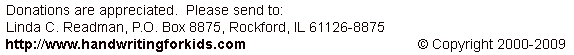Addition: Level 2: Exercise 2 (no carryovers)

Enter your answers in the boxes then click on SUBMIT:

 0+4 1+5 4+2 1+0 3+5 2+6 4+5 2+3 0+9 2+5 1+8 7+0 6+3 5+0 4+4 1+7 4+3 3+5 2+4 3+1 2+0 5+2 2+7 6+0 3+3 6+1 0+0 1+4 8+0 2+1